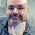### Current Limiting Resistors

Q. For what value of the current limiting resistor will the LED(3.2V, 20 mA) light up safely, when connected to an 18V DC power supply?

The Solution-
If you found the resistor to be 740 Ohms- 1/2 Watt, congrats! If not, continue reading below to understand how to go about such problems.

Firstly, notice that the circuit is simple one closed loop without any parallel paths.

We are given the fact that LED is rated 20mA at 3.2 V.
So the current flowing in the entire circuit would be 20 mA as there is only one path through which current can flow.

Let's recall a simplified version of Kirchoff's loop rule, discussed in the previous question.

GOLDEN RULE- More simply, the sum of the emfs (voltages supplied by sources) in any closed loop is equivalent to the sum of the voltage drops across components in that loop.

As we know that the potential drop across the LED should be 3.2 V, we can form a closed loop equation going by the rule stated above-

So, the voltage drop across the resistor must be 14.8 V.

Or in simpler words, " The LED needs to be fed with 3.2V and the battery provides it with 18V, so the resistor must eat up the extra 14.8V "

Therefore, the value of the current limiting resistor must be around 740 Ohms.

The power rating for the resistor can be calculated as
P=I2R

P= (20 x 10-3)2 x 740 Watts

P=0.296 Watts

For this a 1/4 (or 0.25) Watt resistor would get heated up and may eventually start burning. A 1/2 Watt resistor would do the trick!

We can use a standard value resistor of 820 Ohms which is available at the stores.

After doing the math, all you need to do is get one of these-Hope this article equipped you with the basics of Kirchhoff's Epic Laws!

1.You make figuring electronic problems way to complicated. Keep it simple without thinking like an airhead Engineer.

1.So, go create your own blog, and do it your way. I don't see a reference to your blog, so you obviously haven't bothered - so let this guy do his thing in peace. I find no fault in his instructional method. (For reference, I teach computer science.)

2.Thanks pal, I finally understood, how to calculate resistor. Really great and don't bother with anyone ho knows it long time.